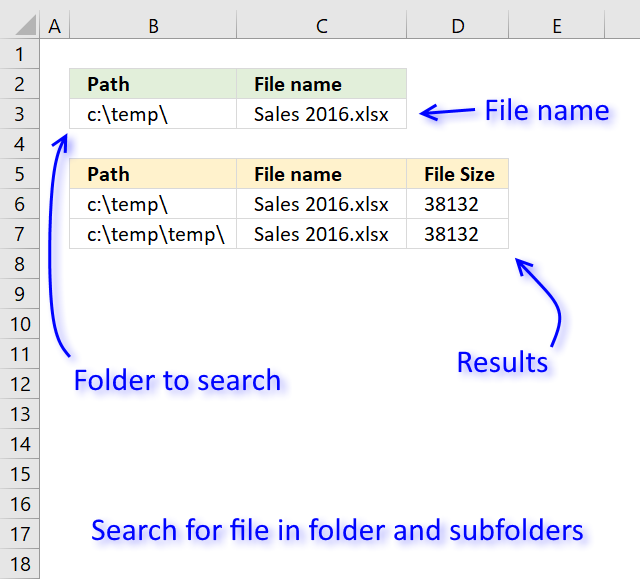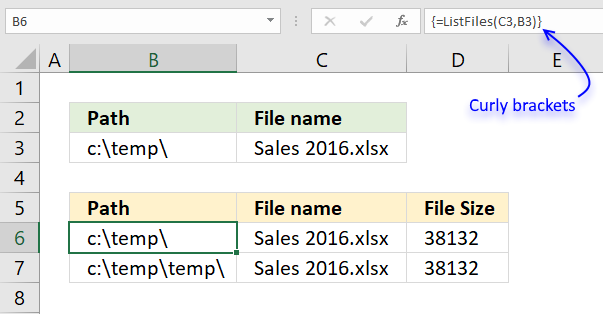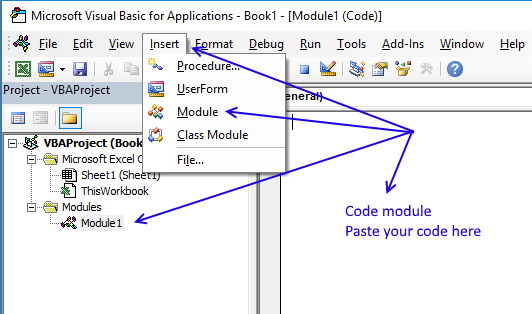Author: Oscar Cronquist Article last updated on December 10, 2019The image above demonstrates a user-defined function in cell range B6:D7 that allows you to search a folder and subfolders for a file name. It returns the exact path to the file, file name, and the file size.

A user defined function is a custom function that you can build yourself if none of Excel's built-in functions works for you.

Array formula in cell range B6:D7:

=ListFiles(C3,B3)

To enter an array formula, select cell range B6:D7. Type the formula and then press and hold CTRL + SHIFT simultaneously, now press Enter once. Release all keys.The formula bar now shows the formula with a beginning and ending curly bracket telling you that you entered the formula successfully. Don't enter the curly brackets yourself.

### User Defined Function Syntax

ListFiles(filename, path)

### Arguments

 filename Required. The filename you are looking for. path Required. The full path to the folder you want the UDF to begin searching in. The function will also search in all subfolders of this folder.

### VBA Macro

There are actually two user-defined functions in order to search all subfolders and a variable that needs to be located at the very top of your workbook's code module.

```Public temp() As String
```

Here is the first UDF, it makes sure that the result doesn't contain any error values.

```Function ListFiles(FileName As String, FolderPath As String)
Dim k As Long, i As Long
ReDim temp(2, 0)
If Right(FolderPath, 1) <> "\" Then
FolderPath = FolderPath & "\"
End If
Recursive FileName, FolderPath

If k < UBound(temp, 2) Then
Else
For i = UBound(temp, 2) To k
ReDim Preserve temp(UBound(temp, 1), i)
temp(0, i) = ""
temp(1, i) = ""
temp(2, i) = ""
Next i
End If
ListFiles = Application.Transpose(temp)
ReDim temp(0)
End Function
```

The second UDF is recursive meaning that a new instance of this UDF is created for each subfolder.

```Function Recursive(FileName As String, FolderPath As String)
Dim Value As String, Folders() As String
Dim Folder As Variant, a As Long
ReDim Folders(0)
If Right(FolderPath, 2) = "\\" Then Exit Function
Value = Dir(FolderPath, &H1F)
Do Until Value = ""
If Value = "." Or Value = ".." Then
Else
If GetAttr(FolderPath & Value) = 16 Then
Folders(UBound(Folders)) = Value
ReDim Preserve Folders(UBound(Folders) + 1)
Else
If Value = FileName Then
temp(0, UBound(temp, 2)) = FolderPath
temp(1, UBound(temp, 2)) = Value
temp(2, UBound(temp, 2)) = FileLen(FolderPath & Value)
ReDim Preserve temp(UBound(temp, 1), UBound(temp, 2) + 1)
End If
End If
End If
Value = Dir
Loop
For Each Folder In Folders
Recursive FileName, FolderPath & Folder & "\"
Next Folder
End Function
```

### Where to put the code?

Follow these steps to insert the code into your workbook.

1. Copy macro.
2. Go to VB Editor (Alt+F11).3. Press with mouse on "Insert" on the top menu.
4. Press with left mouse button on "Module" to insert a module into the workbook.
5. Paste macro to the code module.
6. Exit VB Editor.
7. Save the workbook as a macro-enabled workbook (*.xlsm).
If you don't the macro will be gone the next time you open the workbook.

### Get the Excel fileCheck-if-file-exists-in-folder-or-subfolders-VBA.xlsm

## Search for a file in folder and subfolders using the FSO objectThe following User defined Function, shown in the image above, allows you to search through folders and subfolders for a specified file name.

The first UDF above utilizes the DIR function that sometimes returns error 52 which translates to "Bad file name or number", the FSO object seems to handles this better.

VBA code

```Public temp() As String

Function ListFiles(FileName As String, FolderPath As String)
Dim k As Long, i As Long
ReDim temp(2, 0)
If Right(FolderPath, 1) <> "\" Then
FolderPath = FolderPath & "\"
End If
Recursive FileName, FolderPath

If k < UBound(temp, 2) Then
Else
For i = UBound(temp, 2) To k
ReDim Preserve temp(UBound(temp, 1), i)
temp(0, i) = ""
temp(1, i) = ""
temp(2, i) = ""
Next i
End If
ListFiles = Application.Transpose(temp)
ReDim temp(0)
End Function
```
```Function Recursive(FileName As String, FolderPath As String)
Dim objFSO As Object
Dim Value As String, Folders() As String
Dim Folder As Variant, a As Long
ReDim Folders(0)

Set objFSO = CreateObject("Scripting.FileSystemObject")
Set objFolder = objFSO.GetFolder(FolderPath)

If Right(FolderPath, 2) = "\\" Then Exit Function

For Each objFile In objFolder.Files
If objFile.Name = FileName Then

temp(0, UBound(temp, 2)) = FolderPath
temp(1, UBound(temp, 2)) = objFile.Name
temp(2, UBound(temp, 2)) = objFile.Size
ReDim Preserve temp(UBound(temp, 1), UBound(temp, 2) + 1)

End If

Next objFile

For Each objFolder In objFolder.Subfolders

Folders(UBound(Folders)) = objFolder.Name
ReDim Preserve Folders(UBound(Folders) + 1)

Next objFolder

For Each Folder In Folders

On Error Resume Next
Recursive FileName, FolderPath & Folder & "\"
On Error GoTo 0

Next Folder

End Function
```

### Get the Excel fileCheck-if-file-exists-in-folder-or-subfolders-VBA-FSO.xlsm